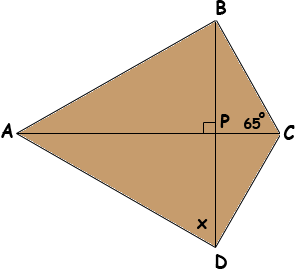Hello, I hope you can help me, The first attachment gives the problem with the figure. The 2nd attachment gives a clearer drawing of the figure by me drawing it. In case you can't read the problem, I'll repeat it here: In the quadrilateral above, AB=AC=AD. If angle BCA = 65, then x=??? The answer is 40, but I can't get it. I know angle BCA=angle ACD=65 degrees and I know that angle ADC=25 degrees. And then for some reason which I do NOT understand, you're supposed to do 65-25=40.Why? Thank you for your help .I appreciate. Hi Jeff, I redrew the diagram and labeled the point P where the line segments AC and BD meet.I don't see how you know that angle ACD=65 degrees or that angle ADC=25 degrees. I see that angle BCA = angle CBA = 65 degrees and, since angle BPC is a right angle, angle CBP = 25 degrees. My proof hinges on the fact that triangles BAP and PAD are congruent. Can you see why? Penny